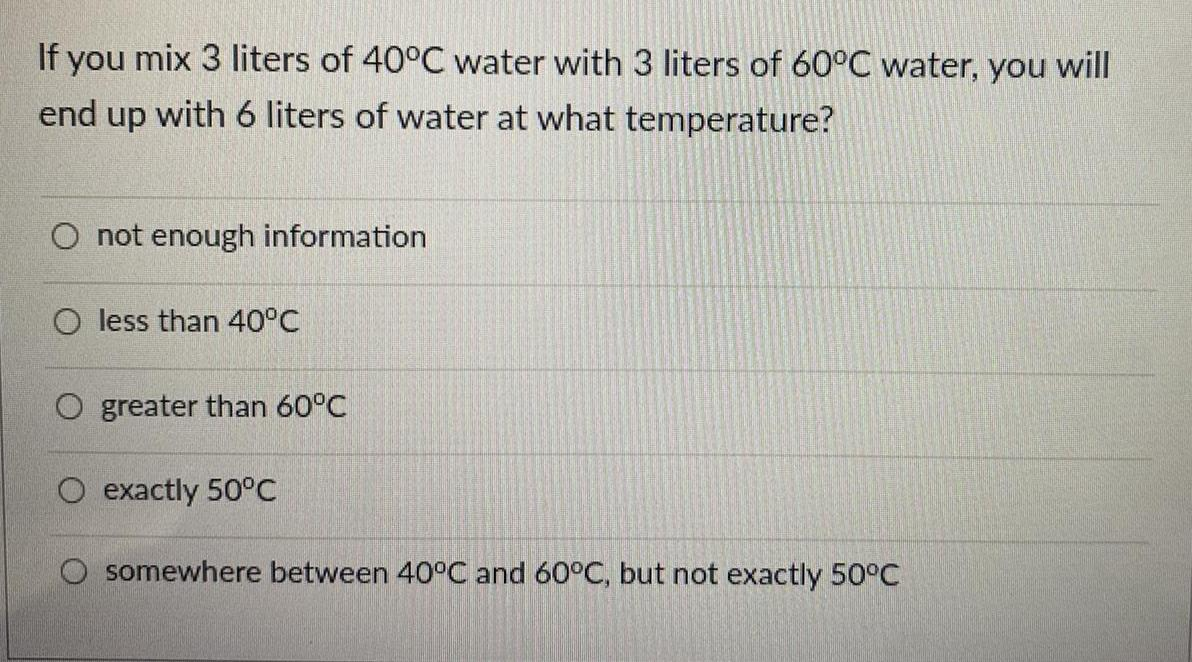Physics
Calorimetry
If you mix 3 liters of 40°C water with 3 liters of 60°C water, you will end up with 6 liters of water at what temperature? A. not enough information B. less than 40°C C. greater than 60°C D. exactly 50°C E. somewhere between 40°C and 60°C, but not exactly 50°C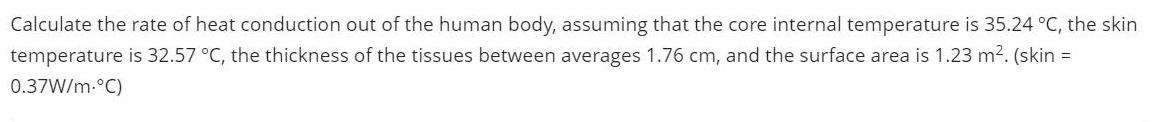Physics
Calorimetry
Calculate the rate of heat conduction out of the human body, assuming that the core internal temperature is 35.24 °C, the skin temperature is 32.57 °C, the thickness of the tissues between averages 1.76 cm, and the surface area is 1.23 m². (skin = 0.37W/m.°C)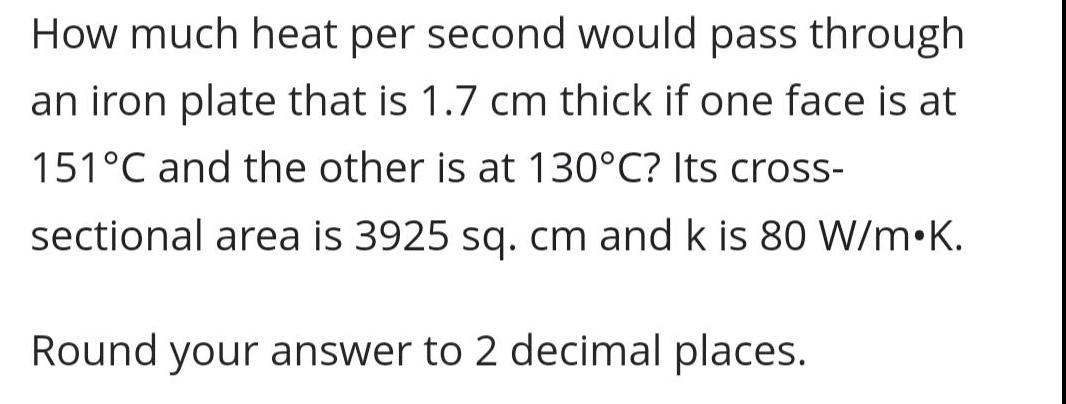Physics
Calorimetry
How much heat per second would pass through an iron plate that is 1.7 cm thick if one face is at 151°C and the other is at 130°C? Its cross- sectional area is 3925 sq. cm and k is 80 W/m.K. Round your answer to 2 decimal places.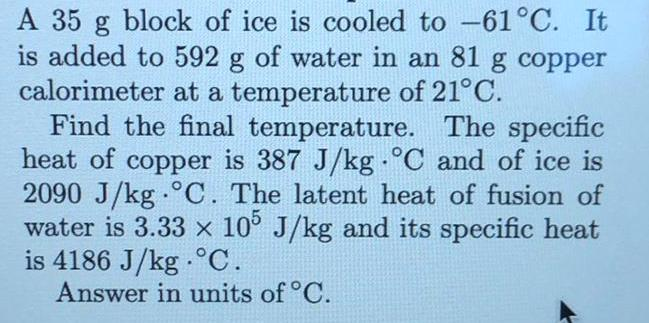Physics
Calorimetry
A 35 g block of ice is cooled to -61°C. It is added to 592 g of water in an 81 g copper calorimeter at a temperature of 21°C. Find the final temperature. The specific heat of copper is 387 J/kg °C and of ice is 2090 J/kg C. The latent heat of fusion of water is 3.33 x 105 J/kg and its specific heat is 4186 J/kg °C. Answer in units of °C.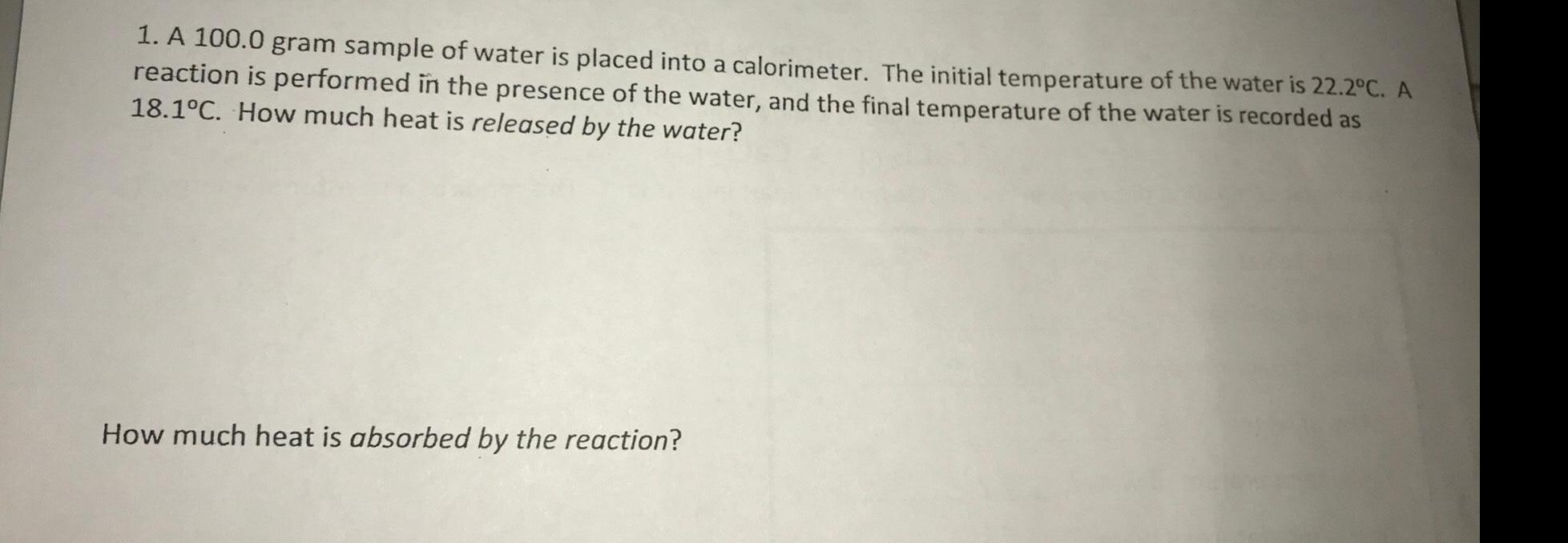Physics
Calorimetry
A 100.0 gram sample of water is placed into a calorimeter. The initial temperature of the water is 22.2°C. A reaction is performed in the presence of the water, and the final temperature of the water is recorded as 18.1°C. How much heat is released by the water? How much heat is absorbed by the reaction?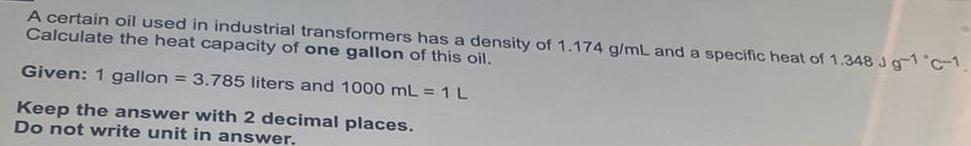Physics
Calorimetry
A certain oil used in industrial transformers has a density of 1.174 g/mL and a specific heat of 1.348 Jg-1 °C-1. Calculate the heat capacity of one gallon of this oil. Given: 1 gallon = 3.785 liters and 1000 mL = 1 L Keep the answer with 2 decimal places. Do not write unit in answer.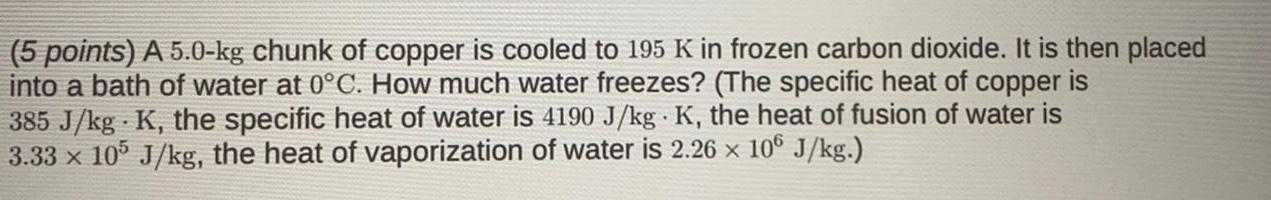Physics
Calorimetry
A 5.0-kg chunk of copper is cooled to 195 K in frozen carbon dioxide. It is then placed into a bath of water at 0°C. How much water freezes? (The specific heat of copper is 385 J/kg K, the specific heat of water is 4190 J/kg K, the heat of fusion of water is 3.33 x 105 J/kg, the heat of vaporization of water is 2.26 x 106 J/kg.)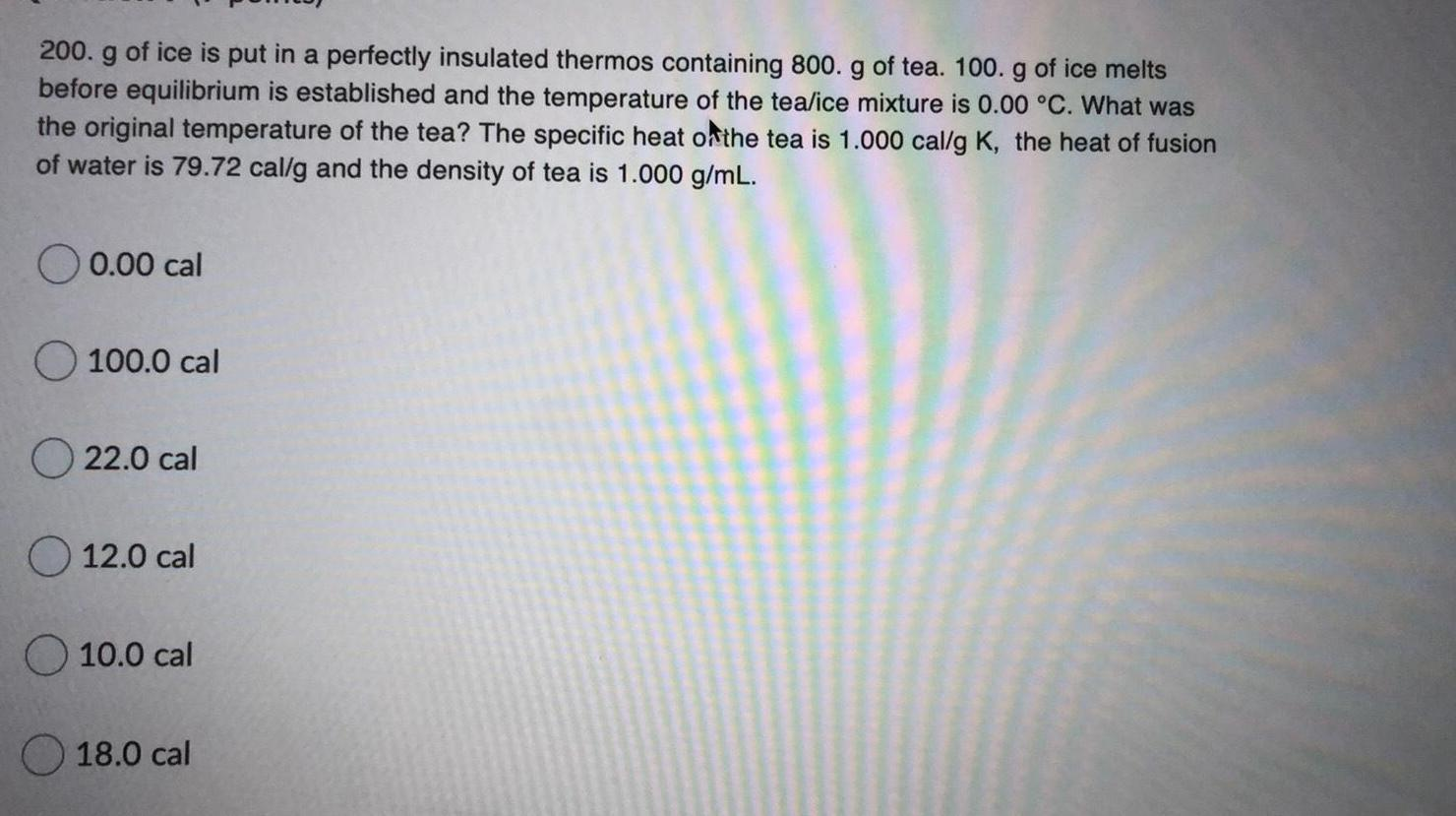Physics
Calorimetry
200. g of ice is put in a perfectly insulated thermos containing 800. g of tea. 100. g of ice melts before equilibrium is established and the temperature of the tea/ice mixture is 0.00 °C. What was the original temperature of the tea? The specific heat of the tea is 1.000 cal/g K, the heat of fusion of water is 79.72 cal/g and the density of tea is 1.000 g/mL. 0.00 cal 100.0 cal 22.0 cal 12.0 cal 10.0 cal 18.0 cal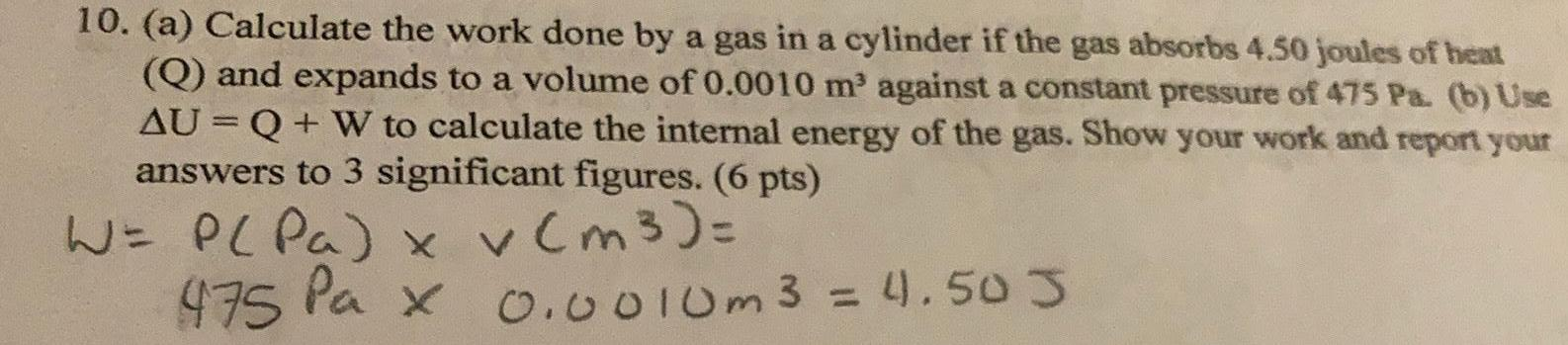Physics
Calorimetry
(a) Calculate the work done by a gas in a cylinder if the gas absorbs 4.50 joules of heat (Q) and expands to a volume of 0.0010 m³ against a constant pressure of 475 Pa. (b) Use ΔU = Q + W to calculate the internal energy of the gas. Show your work and report your answers to 3 significant figures.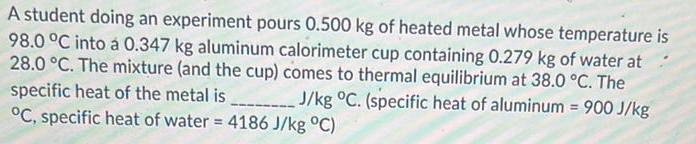Physics
Calorimetry
A student doing an experiment pours 0.500 kg of heated metal whose temperature is 98.0 °C into á 0.347 kg aluminum calorimeter cup containing 0.279 kg of water at 28.0 °C. The mixture (and the cup) comes to thermal equilibrium at 38.0 °C. The specific heat of the metal is J/kg °C. (specific heat of aluminum = 900 J/kg °C, specific heat of water = 4186 J/kg °C)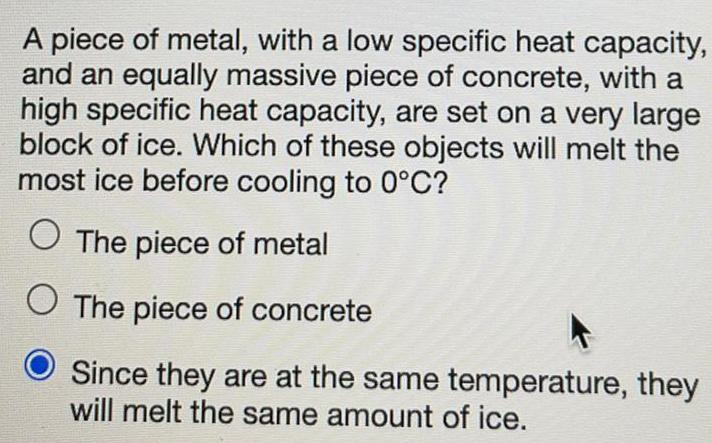Physics
Calorimetry
A piece of metal, with a low specific heat capacity, and an equally massive piece of concrete, with a high specific heat capacity, are set on a very large block of ice. Which of these objects will melt the most ice before cooling to 0°C? The piece of metal The piece of concrete Since they are at the same temperature, they will melt the same amount of ice.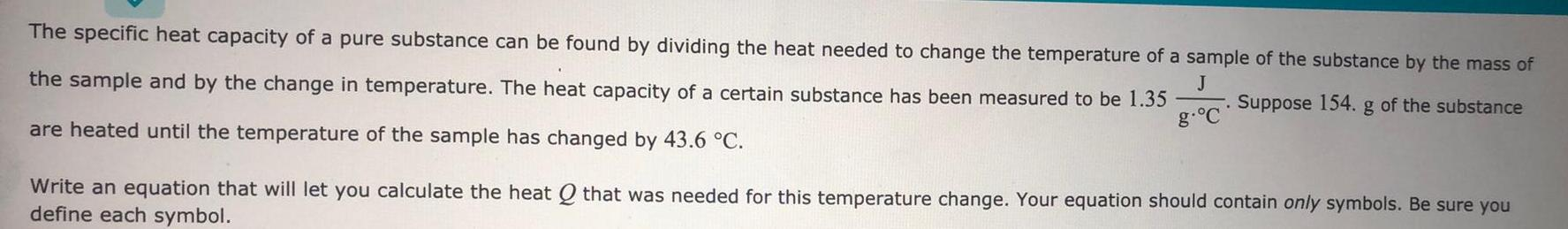Physics
Calorimetry
The specific heat capacity of a pure substance can be found by dividing the heat needed to change the temperature of a sample of the substance by the mass of the sample and by the change in temperature. The heat capacity of a certain substance has been measured to be 1.35J/g.°C Suppose 154. g of the substance are heated until the temperature of the sample has changed by 43.6 °C. Write an equation that will let you calculate the heat Q that was needed for this temperature change.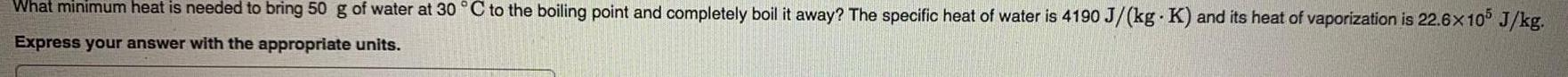Physics
Calorimetry
What minimum heat is needed to bring 50 g of water at 30 °C to the boiling point and completely boil it away? The specific heat of water is 4190 J/(kg. K) and its heat of vaporization is 22.6x10^5 J/kg. Express your answer with the appropriate units.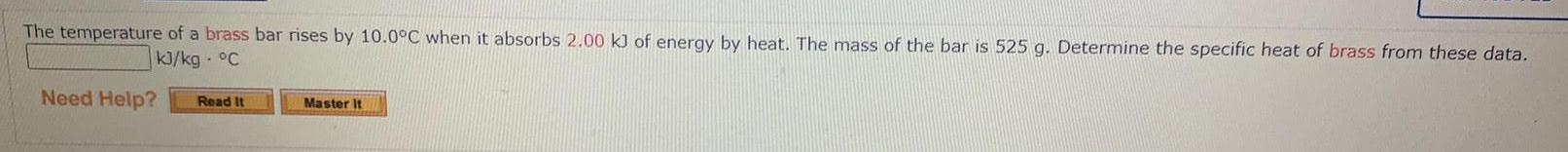Physics
Calorimetry
The temperature of a brass bar rises by 10.0°C when it absorbs 2.00 kJ of energy by heat. The mass of the bar is 525 g. Determine the specific heat of brass from these data.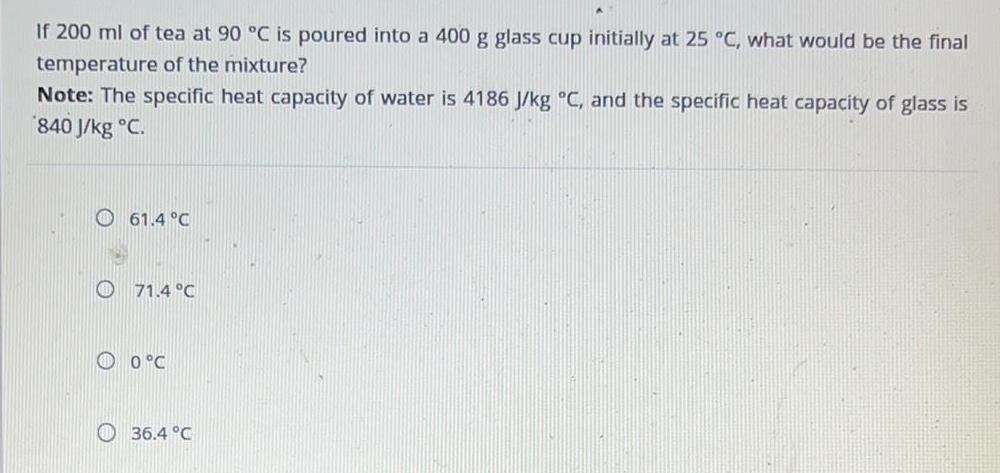Physics
Calorimetry
If 200 ml of tea at 90 °C is poured into a 400 g glass cup initially at 25 °C, what would be the final temperature of the mixture? Note: The specific heat capacity of water is 4186 J/kg °C, and the specific heat capacity of glass is 840 J/kg °C. 61.4 °C 71.4 °C 0°C 36.4 °CPhysics
Calorimetry
you hammer a nail into wood, the nail heats up. 30 Joules of energy was absorbed by a 5-g nail as it was hammered into place. How much does the nail's temperature increase (in °C) during this process? (The specific heat capacity of the nail is 450 J/kg-°C, and round to 3 significant digits.